## Duffing Differential Equation

The most general forced form of the Duffing equation is(1)

If there is no forcing, the right side vanishes, leaving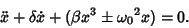(2)

If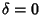and we take the plus sign,(3)

This equation can display chaotic behavior. For, the equation represents a hard spring,'' and for, it represents a soft spring.'' If, the phase portrait curves are closed. Returning to (1), take,,, and use the minus sign. Then the equation is(4)

(Ott 1993, p. 3). This can be written as a system of first-order ordinary differential equations by writing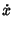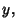(5)(6)

The fixed points of these differential equations(7)

so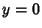, and(8)

giving. Differentiating,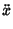(9)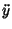(10)(11)

Examine the stability of the point (0,0):(12)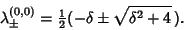(13)

But, sois real. Since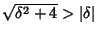, there will always be one Positive Root, so this fixed point is unstable. Now look at (, 0).(14)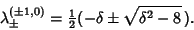(15)

For,, so the point is asymptotically stable. If,, so the point is linearly stable. If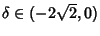, the radical gives an Imaginary Part and the Real Part is, so the point is unstable. If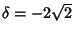,, which has a Positive Real Root, so the point is unstable. If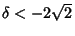, then, so both Roots are Positive and the point is unstable. The following table summarizes these results.asymptotically stablelinearly stable (superstable)unstable

Now specialize to the case, which can be integrated by quadratures. In this case, the equations become(16)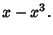(17)

Differentiating (16) and plugging in (17) gives(18)

Multiplying both sides by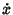gives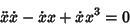(19)(20)

so we have an invariant of motion,(21)

Solving forgives(22)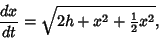(23)

so(24)

Note that the invariant of motionsatisfies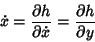(25)(26)

so the equations of the Duffing oscillator are given by the Hamiltonian System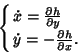(27)

References

Ott, E. Chaos in Dynamical Systems. New York: Cambridge University Press, 1993.